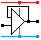Home

rOm
Quest
Glossary

Random
Page
Search
Site
Lush
Sim
Class
Subject
Images

Help
FAQ
Sign
Up
Log
In
 GCSE     Op Amp     Amplifier System     >Comparator<     Gain     Gain Bandwidth     Inverting     Non-Inverting     Power     Summing

# Op Amp Comparator

Site for Eduqas/WJEC - Go to the AQA site.

GCSE    Op Amps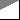Questions 0 to 30   -->  View All

Operational amplifiers have a very high open loop gain.
Tiny differences in the two input voltages cause the output to saturate high or low.
If V+ is higher than V- the output will be high ( low otherwise ).

## Comparator Behaviour

• Comparator subsystems compare two input voltages.
• The comparator output is always HIGH or LOW.
• This happens because the Op Amp has a very high open loop gain.
• Very small changes in the input cause the op amp to saturate low or high.
• A comparator is also a One Bit Analogue to Digital Converter (ADC).
• A comparator compares two ANALOGUE input voltages.
• These are usually a reference voltage and a signal from a sensor.
• The output goes LOW or HIGH when the signal input crosses the reference voltage. This is a DIGITAL signal.
• For a real-life op' amp' this gain will be between 105 and 107.
• This means that a potential difference between V1 and V2 of only a few microvolts is sufficient to saturate the op' amp'.

## Inverting and Non-Inverting Inputs

• Inverting: If the inverting input voltage rises above the other input, the op amp output voltage drops low.
• Non-Inverting: If the non-inverting input voltage rises above the other input, the op amp output voltage rises high.
• With the very high open loop gain of the op amps, these output changes are very sudden and large.
• If V1 is greater than V2 then Vout will go high (close to the + supply voltage)
• If V1 is less than V2 then Vout will go low (close to the - supply voltage).
• It is WRONG and confusing to call these the minus and plus inputs. Please use their correct names.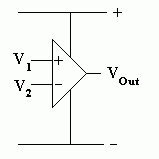## Simple Comparator Example

### Specification

Turn on the LEDs when it gets dark.

• Run on 10 to 15 Volts. Check this with a multimeter.
• Turn on the LEDs when the light level drops below 100 Lux. Check this with a Lux meter.
• Work correctly at 100 Lux at all voltages between 10 and 15 Volts. Use the Lux meter and multimeter to conduct this test.

### How it Works

• MODULE 1
• R1 and R2 form a voltage divider.
• Since the resistors are equal, the voltage is divided equally and the potential at A is about 6V.
• MODULE 2
• R3 and the LDR form a potential divider.
• In the light, the LDR resistance is lower so the voltage at B rises.
• In the dark, the LDR resistance increases so the voltage at B drops.
• MODULE 3
• The 741 operational amplifier chip is being used as a comparator.
• The open loop gain of the op amp is very large (more than 100000).
• This means that tiny differences in the input voltages cause large changes in the op amp output.
• In bright light, the voltage at B is greater than the voltage sat A so the output of the op amp (C) is low (close to 0V). The op amp will be saturated.
• In dim light, the voltage at B is less than the voltage at A so the output of the op amp (C) is high (close to +12V). The op amp will be saturated.
• MODULE 4
• In the dark, the voltage at C is high (+12V) so about 10mA will flow through R4 and the LEDs will be lit.
• In bright light, the voltage at C is low (0V) so no current flows through the LEDs.
• Why Two LEDs?
• An ideal op amp would be able to produce output voltages of +12V and 0V.
• Real life op amps are not so good. They might only manage +9V high or +3V low.
• The low output voltage would still be enough to light a single LED even when it was not supposed to be.
A fault like this could be fatal in an aircraft "Your Engine is On Fire" alert.
• By using two LEDs, each of which needs about 2V to light, four volts are needed to light the LEDs.
This ensures that the LEDs go off when they are supposed to.

### Circuit Diagram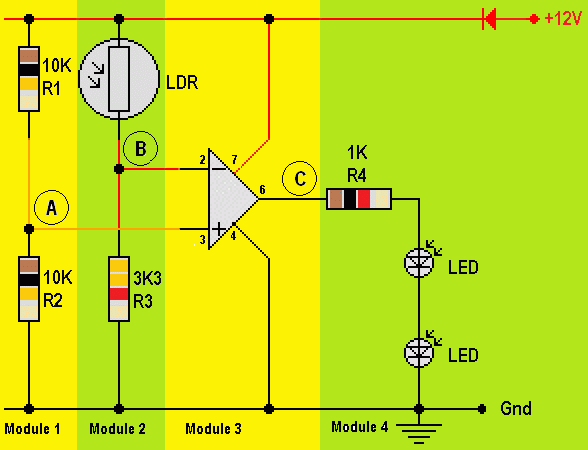### Layout Diagram## Use a comparator to control a MOSFET switch driver circuit.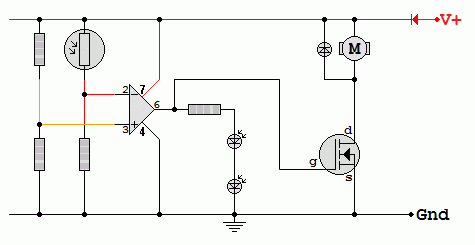## Simulation

Subject Name     Level     Topic Name     Question Heading     First Name Last Name Class ID     User ID

 CloseEscapeX Q: qNum of last_q     Q ID: Question ID         Score: num correct/num attempts         Date Done

Question Text

image url

Help Text
Debug

• You can attempt a question as many times as you like.
• If you are logged in, your first attempt, each day, is logged.
• To improve your scores, come back on future days, log in and re-do the questions that caused you problems.
• In the grade book, you can delete your answers for a topic before re-doing the questions. Avoid deleting unless you intend re-doing the questions very soon.